# Composite Thermal Control Systems with Phase Change Material in Metal Foam for Lithium Batteries Cooling

Composite Thermal Control Systems with Phase Change Material in Metal Foam for Lithium Batteries Cooling

Bernardo Buonomo | Oronzio MancaFerdinando Menale Francesco Moriello Sergio Nardini

Dipartimento di Ingegneria, Università degli Studi della Campania “L. Vanvitelli”, Real Casa dell’Annunziata, Via Roma 29, Aversa, Italy

Corresponding Author Email:
oronzio.manca@unicampania.it
Page:
157-165
|
DOI:
https://doi.org/10.18280/ti-ijes.652-403
22 March 2021
|
Accepted:
26 May 2021
|
Published:
30 July 2021
| Citation

OPEN ACCESS

Abstract:

Electric cars, in addition to representing an ecological solution, represent a turning point in terms of renewal for the world economy. One of the main problems of electric cars is given by the thermal control of their batteries, as below and above a certain temperature range, they abruptly decrease the range of the vehicle, creating inconvenience to the owners of such cars.

The thermal control of lithium batteries for electric cars must therefore take into account both the problems of thermal increase due to the operation of the battery itself, and the climatic conditions outside the vehicle that impact, if above a certain range, negatively on the performance of the car, decreasing both the range and the life of the battery.

In this study, an attempt is made to control the temperature peaks due to the operation of the battery itself to this end, a thermal control system with metal foam phase change material is studied in order to evaluate the heat transfer behavior for use in the cooling of lithium batteries. A two-dimensional model is considered to numerically study the thermal control with different charge and discharge cycles. The battery is simulated as a wall-mounted heat flow. Thermal control is achieved by means of an inner layer of copper foam and phase change material, PCM (paraffin) and the outer surfaces are cooled by convective flow. The governing equations, written assuming local thermal equilibrium for the metal foam, are solved by the finite volume method using the commercial Ansys-Fluent code. Several cases are simulated for different values of external convective heat transfer coefficient. Results, performed for metal foams with different PPIs and porosities, are provided in terms of temperature fields and liquid fraction, heat transfer behaviors such as surface temperature profiles as a function of time, and temperature distributions along the outer surface of the battery for the different cases. In addition, some comparisons with pure PCM within the thermal control system are provided to show the advantages of the composite thermal control system with PCM within the metal foam.

The results obtained show that the best solution among those studied is given by the use of copper foams filled with paraffin PCM.

Keywords:

Li-ion battery, PCM, copper foam, thermal control

1. Introduction

The serious environmental problems of recent decades, mainly due to pollutants emitted by fossil fuels from internal combustion engine vehicles, are driving research into increasingly sustainable solutions based on the use of renewable energy sources. One of the most promising solutions to curb pollution in built-up areas, and one that is also revolutionizing the world economy, is electric vehicles, which are slowly making their mark both commercially and as a consumer choice.

This type of vehicle, unlike those equipped with the classic propulsion with fossil fuels, uses an electric motor that uses as primary energy the chemical energy that is stored through rechargeable batteries and then made available to the engine as electrical energy.

Compared to the classic internal combustion engine, electric vehicles are more energy efficient, but have some issues due to the use of batteries. These vehicles use lithium-ion batteries, but they require long recharging times with low battery life and have serious thermal problems that can make their use dangerous.

Research on thermal control of this type of batteries is essential to avoid both health hazards and economic damage to the production companies. Over the years, many techniques have been used for thermal control of these devices and one of the most promising and current is the combined use of phase change materials (PCM) and metal foams. Among the main innovators in the use of this thermal control technique is Libeer and his research group  who performed studies for the application of PCM and metal foams for battery management system, concluding that the use of foam with PCM improved the heat transfer of the devices they analyzed. Research done by Lafdi et al.  on the use of metal foams with PCM, showed that larger foam pores result in lower surface temperatures due to high convective flow. Research by Landini et al.  shows that the typical temperature range for the use of this type of battery should be 25-40°C and that the optimal temperature range is 25-30°C. Different models for thermal management of batteries with different geometric shapes have been studied by Safdari et al.  who understood that the best shape to be given is the rectangular one. As shown by Buonomo et al.  the use of PCMs, due to the high latent heat value, allows to delay the achievement of the critical temperature of the battery until the completion of liquefaction and that, the use of metal foams, allow to distribute uniformly the thermal energy in the phase change material avoiding that it, given the low thermal conductivity, behaves as a thermal insulator. A thermal management system with copper and paraffin foams was numerically and experimentally implemented by Kiani et al. , integrated with an active cooling system with alumina nanofluid as cooling fluid. They concluded that the nanofluid effectively postpones the onset of phase transition by increasing the melting time of paraffin. A study on the thermal response of lithium batteries in high discharge rates with a novel TMS combining active and passive methods was conducted by Mashayekhi et al. . Their results show that passive cooling was inefficient in keeping the battery temperature below the safe limit at high discharge rates, while the active hybrid system showed adequate thermal performance under the same conditions. Nine passive battery thermal management systems (BTMS) based on paraffin as pure PCM and copper foam as conductive additive, but with nine different amounts (from 1 to 9 vol%), were numerically simulated by Ranjbaran et al.  to reveal the role of additive content. Their results showed that the addition of metal foam significantly affects the time evolution of the PCM liquid fraction. The heat transfer and phase change flux inside a lithium battery enclosure with a copper foam and paraffin PCM are numerically studied by Veismoradi et al. . Their results demonstrate that for high heat pulse powers, the melt volume fraction (MVF) rises and the heat sink will have higher efficiency. For a relatively strong heat pulse, the efficiency was improved by about seven times.

El Idi et al.  fabricated a composite of metal foams and paraffin by the vacuum impregnation method, modeling the thermal conductivity of the composite as a function of the foam structure, the thermal conductivity of the metal foam, and the thermal conductivity of the PCM.  The results demonstrate a high impact of the thermal conductivity of the metal foam and a lower effect of pore size on the effective thermal conductivity.

Few studies still exist in the literature on the combination of metal foams with phase change materials (PCMs) for thermal control of lithium-ion batteries, and this research work seeks to make an original and innovative contribution on this promising combination, which could evolve current thermal control systems for electric vehicle batteries while avoiding the safety issues encountered so far.

In this research work, a passive system for cooling lithium batteries for electric vehicles has been implemented. A numerical model has been realized through a system with PCM and copper foams using the porosity enthalpy method. The simulations performed were obtained through the use of Ansys Fluent and the results were reported in terms of average temperatures and melting time achieved.

2. Physical Model and Governing Equations

The physical domain consists of a zone where, based on various simulations, air has been introduced, copper foam at 40 PPI with ε=0.934, the RT42 PCM melting at about 42°C  and the copper foam filled by the PCM.

The operating temperature of the lithium batteries is expected to remain around 318 K .

Figure 1 shows the 2D domain used in this study where the dimensions, obtained from a commercial electric battery, are L=310mm, H=40 mm.

## 1.pngFigure 1. Physical Domain

The properties of the materials and the copper foam have been reported in Table 1 and Table 2.

The enthalpy-porosity method  is used to describe the melting phenomenon of PCM, where the solid-liquid interface is not drawn explicitly but the method defines a mixed solid-liquid region that represents the "pseudo" porous zone that is the liquid fraction. The liquid fraction β has a value of 0 when the zone is totally solid, 1 when it is totally liquid, and between 0 and 1 for the mixed region.

Table 1. Properties of materials

 Thermal Properties Paraffin RT42 Copper Air Density [kg/m3] 820 8978 1.225 Specific Heat [J/kg K] 2000 381 1006.4 Thermal Conductivity [W/m K] 0.20 387.6 0.0242 Dynamic Viscosity [kg/m s] 0.020 - 1.789e-5 Thermal expansion coefficient [1/K] 0.0001 - 0.00333 Melting Heat [J / kg] 165000 - - Solidus Temperature [K] 311.15 - - Liquidus Temperature [K] 316.15 - -

Table 2. Properties of copper foams 

 Cu-40-6.6 Number of pores per inch, PPI 40 Relative density, ρR(%) 6.6 Porosity, ε (–) 0.934 Fiber thickness, t (mm) 0.262 Fiber lenght l (mm) 1.109 Surface are per unit of volume, asv(m2m-3) 1635 Permeability, K (m2) ×107 0.44 Inertia coefficient CF 0.060

$\left\{\begin{array}{l}\beta=0 \quad \text { for } T<T_{\text {solidus }} \\ \beta=\frac{T-T_{\text {solidus }}}{T_{\text {liquidus }}-T_{\text {solidus }}} \quad \text { for } T_{\text {solidus }}<T<T_{\text {liquidus }} \\ \beta=1 \quad \text { for } \quad T>T_{\text {liquidus }}\end{array}\right.$      (1)

In (1) T is the local temperature of the cell, Tliquidus is the upper temperature at which the domain is totally liquid, and Tsolidus is the temperature below which it is totally solid. The solid part of the mixed zone is modeled by adding a source term in the momentum equation. The metal foam is modeled with the Darcy-Forchheimer extended Brinkman model because it behaves as a porous medium; The gravitational acceleration is along the y-axis and the Boussinesq approximation is considered to account for the buoyancy force due to natural convection. The thermal interaction between the metal foam and the flows is modeled with the LTE assumption, where a unique local temperature function is defined between the porous medium and the fluid flow. The reference equations are as follows:

-PCM

$\frac{\partial u_{p c m}}{\partial x}+\frac{\partial v_{p c m}}{\partial y}=0$     (2)

$\frac{\rho_{p c m}}{\varepsilon}\left(\frac{\partial u_{p c m}}{\partial t}+\frac{u_{p c m}}{\varepsilon} \frac{\partial u_{p c m}}{\partial x}+\frac{v_{p c m}}{\varepsilon} \frac{\partial u_{p c m}}{\partial y}\right)$$=-\frac{\partial p_{p c m}}{\partial x}+\frac{\mu_{p c m}}{\varepsilon}\left(\frac{\partial^{2} u_{p c m}}{\partial x^{2}}+\frac{\partial^{2} u_{p c m}}{\partial y^{2}}\right)+S_{x} (3) \frac{\rho_{p c m}}{\varepsilon}\left(\frac{\partial v_{p c m}}{\partial t}+\frac{u_{p c m}}{\varepsilon} \frac{\partial v_{p c m}}{\partial x}+\frac{v_{p c m}}{\varepsilon} \frac{\partial v_{p c m}}{\partial y}\right)$$=-\frac{\partial p_{p c m}}{\partial y}+\frac{\mu_{p c m}}{\varepsilon}\left(\frac{\partial^{2} v_{p c m}}{\partial x^{2}}+\frac{\partial^{2} v_{p c m}}{\partial y^{2}}\right)+S_{y}$      (4)

$\left[(1-\varepsilon)(\rho c)_{m f}+\varepsilon(\rho c)_{p c m}\right]$$\left(\frac{\partial T_{p c m}}{\partial t}+u_{p c m} \frac{\partial T_{p c m}}{\partial x}+v_{p c m} \frac{\partial T_{p c m}}{\partial y}\right)=$$=k_{e f f \mid p c m}\left(\frac{\partial^{2} T_{p c m}}{\partial x^{2}}+\frac{\partial^{2} T_{p c m}}{\partial y^{2}}\right)-\varepsilon \rho_{p c m} H_{L} \frac{\partial \beta}{\partial t}$      (5)

-Air

$\frac{\partial u_{\text {air }}}{\partial x}+\frac{\partial v_{\text {air }}}{\partial y}=0$      (6)

$\frac{\rho_{a i r}}{\varepsilon}\left(\frac{\partial u_{a i r}}{\partial t}+\frac{u_{a i r}}{\varepsilon} \frac{\partial u_{a i r}}{\partial x}+\frac{v_{a i r}}{\varepsilon} \frac{\partial u_{a i r}}{\partial y}\right)=$$-\frac{\partial p_{a i r}}{\partial x}+\frac{\mu_{a i r}}{\varepsilon}\left(\frac{\partial^{2} u_{a i r}}{\partial x^{2}}+\frac{\partial^{2} u_{a i r}}{\partial y^{2}}\right) (7) \frac{\rho_{\text {air }}}{\varepsilon}\left(\frac{\partial v_{\text {air }}}{\partial t}+\frac{u_{\text {air }}}{\varepsilon} \frac{\partial v_{\text {air }}}{\partial x}+\frac{v_{\text {air }}}{\varepsilon} \frac{\partial v_{\text {air }}}{\partial y}\right)=$$-\frac{\partial p_{\text {air }}}{\partial y}+\frac{\mu_{\text {air }}}{\varepsilon}\left(\frac{\partial^{2} v_{\text {air }}}{\partial x^{2}}+\frac{\partial^{2} v_{\text {air }}}{\partial y^{2}}\right)+S_{\text {air }}$      (8)

$\left[(1-\varepsilon)(\rho c)_{m f}+\varepsilon(\rho c)_{a i r}\right]$$\left(\frac{\partial T_{a i r}}{\partial t}+u_{a i r} \frac{\partial T_{a i r}}{\partial x}+v_{a i r} \frac{\partial T_{a i r}}{\partial y}\right)=$$=k_{\text {eff } \mid a i r}\left(\frac{\partial^{2} T_{\text {air }}}{\partial x^{2}}+\frac{\partial^{2} T_{\text {air }}}{\partial y^{2}}\right)$     (9)

The heat generation rate simulates a typical battery dissipated power of 5.3 KW.

In the equations, ρ and c are the density and specific heat, respectively; u and v are the velocity in the x and y directions, respectively; ε is the porosity of the metal foam; p is the relative pressure; μ is the dynamic viscosity of the PCM or air; S is the source term; V is the velocity vector of the PCM in the liquid or air phase; and et is the time. The subscripts Lit, pcm, and air referred to the battery, phase change material, and air, respectively. Regarding the local temperature, the LTE approach is used and thus the temperature foam in the PCM domain is equal to the PCM temperature and the same assumption is set in the air domain.

The source terms in the PCM momentum equations are:

$S_{x}=\left(\begin{array}{l}\frac{(1-\beta)^{2}}{\left(\beta^{3}+0.001\right)^{3}} A_{\text {mush }} \\ +\frac{\mu_{p c m}}{K}+\frac{C_{F}}{\sqrt{K}} \rho_{p c m}\left|\vec{V}_{p c m}\right|\end{array}\right) v_{p c m}$      (10)

$S_{y}=\left(\frac{(1-\beta)^{2}}{\left(\beta^{3}+0.001\right)^{3}} A_{m u s h}+\frac{\mu_{p c m}}{K}+\frac{C_{F}}{\sqrt{K}} \rho_{p c m}\left|\vec{V}_{p c m}\right|\right)$

$v_{p c m}+\rho_{p c m} g \gamma_{p c m}\left(T_{p c m}-T_{0}\right)$      (11)

The first term is the Kozeny - Carman term that models the presence of the solid part of the PCM during melting. Amush is the mushy zone constant that takes into account the velocity damping during solidification. Its value is fixed at 105 kg/ (m3s). The second term is the Darcy term where K is the permeability of the porous medium and the third term is the Forchheimer term, where CF is the inertial strength factor.

The details of equation 12 can be found in .

Permeability and resistance coefficients are calculated from:

$\left\{\begin{array}{l}K=0.00073(1-\varepsilon)^{-0.224}\left(\frac{d_{f}}{d_{p}}\right)^{-1.11} d_{p}^{2} \\ C_{F}=0.00212(1-\varepsilon)^{-0.132}\left(\frac{d_{f}}{d_{p}}\right)^{-1.63} \\ \frac{d_{f}}{d_{p}}=1.18 \sqrt{\frac{1-\varepsilon}{3 \pi}}\left(\frac{1}{1-e^{1(1-\varepsilon) / 0.04}}\right) \\ d_{p}=\frac{0.0224}{\omega}\end{array}\right.$       (12)

The last term is the Boussinesq approximation where g is the acceleration modulus of gravity, γpcm is the thermal expansion coefficient of the PCM, and T0 is the operating temperature, set to 310K. keff is the effective thermal conductivity calculated by :

$\left\{\begin{array}{l}K=0.00073(1-\varepsilon)^{-0.224}\left(\frac{d_{f}}{d_{p}}\right)^{-1.11} d_{p}^{2} \\ C_{F}=0.00212(1-\varepsilon)^{-0.132}\left(\frac{d_{f}}{d_{p}}\right)^{-1.63} \\ \frac{d_{f}}{d_{p}}=1.18 \sqrt{\frac{1-\varepsilon}{3 \pi}}\left(\frac{1}{1-e^{1(1-\varepsilon) / 0.04}}\right) \\ d_{p}=\frac{0.0224}{\omega}\end{array}\right.$      (13)

$\left\{\begin{array}{c}\boldsymbol{k}_{\text {eff } \mid \boldsymbol{p c m}}=(\mathbf{1}-\boldsymbol{\varepsilon}) \boldsymbol{k}_{m f}+\boldsymbol{\varepsilon} \boldsymbol{k}_{p c m} \\ \boldsymbol{k}_{\text {eff } \mid \text { air }}=(\mathbf{1}-\boldsymbol{\varepsilon}) \boldsymbol{k}_{m f}+\varepsilon \boldsymbol{k}_{\text {air }}\end{array}\right.$      (14)

3. Numerical Model

Table 3. Grid independence at t=1000s

 Grid Average Temperature (K) ΔT 8x20 395.50 0.1 15x40 395.45 0.05 30x78 395.41 0.01 60x115 395.40 - 120x310 395.40 -

Table 4. Boundary conditions

Physical domain

Zone

Velocity conditions

Thermal conditions

For All cases

AB

u=v=0

$\dot{q}=\dot{\mathrm{q}}_{\mathrm{WALL}}$

For $\mathrm{h}=0$

A-C, B-D

u=v=0

adiabatic: $\frac{\partial T}{\partial x}=0$q

C-D

u=v=0

adiabatic: $\frac{\partial T}{\partial y}=0$

For $\mathrm{h} \neq 0$

A-C

u=v=0

$\mathrm{k}_{\mathrm{TCS}} \frac{\partial \mathrm{T}\left(\mathrm{x}_{\mathrm{A}}, \mathrm{y}\right)}{\partial \mathrm{x}}=\mathrm{h}\left[\mathrm{T}\left(\mathrm{x}_{A}, \mathrm{y}\right)-\mathrm{T}_{\mathrm{ext}}\right]$

$\mathrm{k}_{\mathrm{TCS}} \frac{\partial \mathrm{T}\left(\mathrm{x}_{B}, \mathrm{y}\right)}{\partial \mathrm{x}}=\mathrm{h}\left[\mathrm{T}\left(\mathrm{x}_{B}, \mathrm{y}\right)-\mathrm{T}_{\mathrm{ext}}\right]$

B-D

u=v=0

C-D

u=v=0

$\mathrm{k}_{\mathrm{TCS}} \frac{\partial \mathrm{T}\left(\mathrm{x}, \mathrm{y}_{C}\right)}{\partial \mathrm{y}}=\mathrm{h}\left[\mathrm{T}\left(\mathrm{x}, \mathrm{y}_{C}\right)-\mathrm{T}_{\mathrm{ext}}\right]$

## biao_.png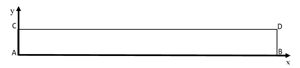Ansys Fluent software  was used to solve the governing equations using the finite volume method. A transient mode with a time interval of 1 s was used. The phenomenon related to coupled velocity-pressure fields has been solved using the SIMPLE algorithm developed by Patankar . A second order wind model is used to treat the convective terms for the momentum and energy equations, and a second order central difference model is used for the discretization of the diffusive terms. For the discretization scheme of the pressure equation, the PRESTO! A transient mode with a step of 0.1 s has been enabled. The convergence errors for the continuity and momentum equation were set to 10-5 and the energy equation was assumed to be 10-8. For the independent mesh solution, five different grids were created using the configuration with copper foams at 40 PPI filled by the PCM, which are: 8x20, 15x40, 30x78, 60x115, 120x310 of which it was decided to use the 60x115 because the calculation costs are satisfactory with precision (Tab. 3).

The boundary conditions have been reported in Table 4.

4. Results and Discussions

In the following research work, simulations were performed to understand the thermal performance of various materials used for thermal control of a modern electric vehicle powered by a lithium-ion battery. Initially, the thermal performance obtained using only air was analyzed, then the analysis of only copper foam 40 PPI (Pores per Inch) with a porosity ε = 0.934, then the analysis of only PCM where a paraffinic phase change material from Rubitherm was used, and finally analysis was conducted on the copper foam filled with a paraffin PCM.

These materials were analyzed both individually and compared to each other to understand the differences. These analyses were conducted with a heat transfer coefficient of h=0, 20, 200 (W/m2K) and they have covered the liquid fractions for only PCM and copper foam filled PCM (Figure 2), the average temperatures of both layers Top Domain and Bottom Domain (Figure 3), average temperatures of the entire domain (Figure 4), the temperature profiles along the bottom layer of the domain (Bottom), and the temperature ranges of only air, only copper foam, and PCM-filled copper foam were obtained for h=20, 200 (W/m2K).

## 2.pngFigure 2. Liquid fraction of domain for only PCM and 40 PPI copper foam filled by PCM h=0, 20, 200 [W/m2K]

## 31.png(a)

## 3.png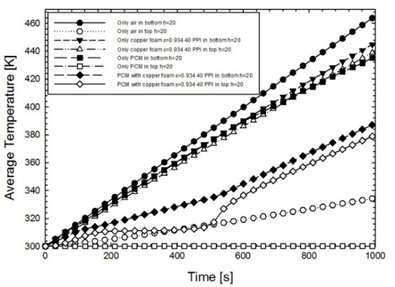(b)

## 33.png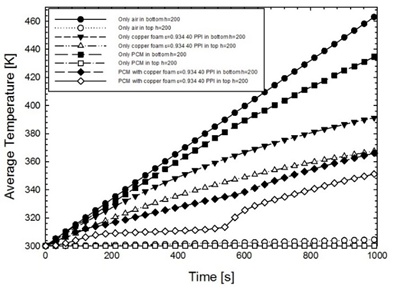(c)

Figure 3. Average temperatures of Bottom and Top layers for only air, 40 PPI copper foam, only PCM, and 40 PPI copper foam filled by PCM (a) h=0 [W/m2K], (b) h=20 [W/m2K], h=100 [W/m2K]

## 41.png(a)

## 42.png(b)

## 43.png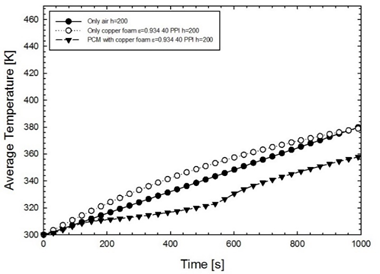(c)

Figure 4. Average temperatures of domain for only air, 40 PPI copper foam, only PCM, and 40 PPI copper foam filled by PCM (a) h=0 [W/m2K], (b) h=20 [W/m2K], h=100 [W/m2K]

## 51.png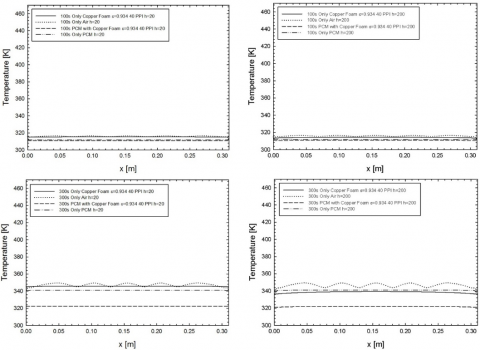## 52.png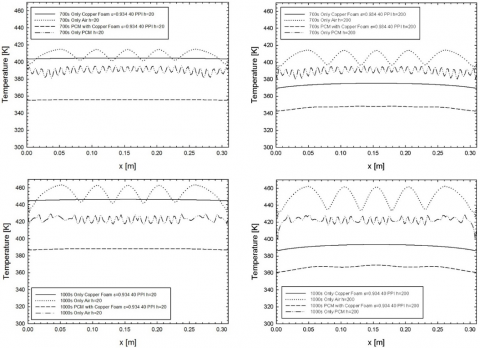Figure 5. Temperature profiles of bottom domain for only air, only 40 PPI copper foam, only PCM, and 40 PPI copper foam filled with PCM

In fact, while with h=0 (W/m2K) the average temperatures of the Top and Bottom layers are close, despite having the highest average temperatures, as the heat transfer coefficient h=20, 200 (W/m2K) increases, the gap between the average temperatures of the two zones increases greatly due to the triggering of convection. The most pronounced differences between the average temperatures of the Top and Bottom domains were found with the use of only PCM, in fact while the average temperature measured on the Bottom layer of the domain, which receives the heat flow, turns out to be at extremely high temperatures and therefore dangerous for the thermal safety parameters of lithium batteries, it completely liquefies, the areas above the Bottom layer up to the Top part of the domain turn out to be at extremely lower average temperatures, not triggering the transition between solid and liquid. The average temperatures obtained using only 40 PPI copper foam ε = 0.934 for the Top and Bottom layers, while less pronounced than previous cases, find extremely high average temperatures.  The best performance regarding average temperatures was obtained with the simulations with PCM-filled copper foams, which in addition to having lower average temperatures than the other cases analyzed, keep the two Top and Bottom layers at closer average temperatures. This happens because as the battery temperature approaches the critical temperature, the PCM begins to melt and thus the temperature tends to be maintained at levels more in tune with the battery safety parameters.As with the simulations conducted with only air, different trends were found depending on the heat transfer coefficient used.

To better understand the thermal effects occurring at the leading edge between the battery and the domain we did, analyses were performed on the temperature profiles for the bottom layer of the domain (Figure 5) for h= 20, 200 (W/m2K), using air only, 40 PPI copper foam only, PCM only, and paraffin-filled copper foam PCM. Again, the lowest temperatures found were achieved through the combined use of PCM and copper foam.

5. Conclusions

From the analysis of the results obtained in this study, as regards the simulations carried out with the only copper foam, it was obtained that the average temperatures of the Top Domain and Bottom Domain layers, even if they have very close average temperature trends, appear to be at temperatures that are too high for thermal control of the batteries. As for the average temperatures of the only air, as the heat exchange coefficient increases, given the triggering of convection, there are too large differences between the Top Domain part and the Bottom Domain part, this means that the battery is almost not affected all of the thermal control based on only air.

The trend of the average temperature for the simulations conducted with the only PCM showed to have the worst trend, that is to have extremely different temperatures between the two layers (Top and Bottom), in fact while the lower layer of the domain, or at the interface with the battery (Bottom Domain), it turns out to have extremely high average temperatures by completely liquefying the PCM layer, the immediately upper layer of the domain turns out to be at extremely lower temperatures at least until the PCM is completely melted. This situation is particularly dangerous for the battery, exposing the vehicle and the user to various dangers. From the simulations obtained, the best trend for the average temperatures was found to be that obtained with the combination of metal foams with PCM, in which the average temperatures of both the Top and Bottom layers and of the entire domain are the lowest and also the differences between the two layers are extremely limited compared to the other cases analyzed and this allows the battery to maintain temperatures close to the safety parameters for a longer time than in the other cases analyzed.

Acknowledgements

This research was partially funded by MIUR (Ministero dell’Istruzione, dell’Università e della Ricerca), grant number PRIN-2017F7KZWS and by Università degli Studi della Campania “Luigi Vanvitelli” with the grant number D.R. n. 138 under NanoTES project - V:ALERE program 2020.

Nomenclature
 Amush Mushy constant [kg/(m3s)] Cf inertial drag factor [-] c specific heat [J/kg K] d diameter [m] g Gravity acceleration [m/s2] HL Latent Heat [J/kg] k thermal conductivity [W/mK] K Permeability [m2] p relative pressure [Pa] S Source term [N/m3] T Local Temperature [K] t Time [s] u”’ heat generation [W/m3] u velocity along x direction [m/s] V Local Velocity module [m/s] v velocity along y direction [m/s] x,y Cartesian directions [m] Greek letters β Liquid Fration [-] ε porosity [-] γ thermal expansion factor [1/K] μ dynamic Viscosity [Pa s] ρ Density [kg/m3] ω Pore Density – Number of pore per Inch [-] Subscript 0 operating air air f fiber Liquidus End melting Temperature mf metal foam p pore pcm phase change material Solidus Start melting Temperature
References

 Libeer, W., Ramos, F., Newton, C., Alipanahrostami, M., Depcik, C., Li, X. (2016). Two-phase heat and mass transfer of phase change materials in thermal management systems. International Journal of Heat and Mass Transfer, 100: 215-223. https://doi.org/10.1016/j.ijheatmasstransfer.2016.04.076

 Lafdi, K., Mesalhy, O., Shaikh, S. (2007). Experimental study on the influence of foam porosity and pore size on the melting of phase change materials. Journal of Applied Physics, 102(8): 083549. https://doi.org/10.1063/1.2802183

 Landini, S., Leworthy, J., O’Donovan, T.S. (2019). A review of phase change materials for the thermal management and isothermalisation of lithium-ion cells. Journal of Energy Storage, 25: 100887. https://doi.org/10.1016/j.est.2019.100887

 Safdari, M., Ahmadi, R., Sadeghzadeh, S. (2020). Numerical investigation on PCM encapsulation shape used in the passive-active battery thermal management. Energy, 193: 116840. https://doi.org/10.1016/j.energy.2019.116840

 Buonomo, B., Ercole, D., Manca, O., Menale, F. (2018). Thermal cooling behaviors of lithium-ion batteries by metal foam with phase change materials. Energy Procedia, 148: 1175-1182. https://doi.org/10.1016/j.egypro.2018.08.024

 Kiani, M., Ansari, M., Arshadi, A.A., Houshfar, E., Ashjaee, M. (2020). Hybrid thermal management of lithium-ion batteries using nanofluid, metal foam, and phase change material: an integrated numerical–experimental approach. Journal of Thermal Analysis and Calorimetry, 141(5): 1703-1715. https://doi.org/10.1007/s10973-020-09403-6

 Mashayekhi, M., Houshfar, E., Ashjaee, M. (2020). Development of hybrid cooling method with PCM and Al2O3 nanofluid in aluminium minichannels using heat source model of Li-ion batteries. Applied Thermal Engineering, 178: 115543. https://doi.org/10.1016/j.applthermaleng.2020.115543

 Ranjbaran, Y.S., Haghparast, S.J., Shojaeefard, M.H., Molaeimanesh, G.R. (2020). Numerical evaluation of a thermal management system consisting PCM and porous metal foam for Li-ion batteries. Journal of Thermal Analysis and Calorimetry, 141(5): 1717-1739. https://doi.org/10.1007/s10973-019-08989-w

 Veismoradi, A., Modir, A., Ghalambaz, M., Chamkha, A. (2020). A phase change/metal foam heatsink for thermal management of battery packs. International Journal of Thermal Sciences, 157: 106514. https://doi.org/10.1016/j.ijthermalsci.2020.106514

 El Idi, M.M., Karkri, M., Kraiem, M. (2021). Preparation and effective thermal conductivity of a Paraffin/Metal Foam composite. Journal of Energy Storage, 33: 102077. https://doi.org/10.1016/j.est.2020.102077

 Rubitherm GmBH, www.rubitherm.de.

 Sparkfan electronic, Lithium Ion Rechargeable Batteries Technical Handbook, [online] Available: https://cdn.sparkfun.com/datasheets/Prototyping/Lithium%20Ion%20Battery%20MSDS.pdf.

 Al-Abidi, A.A., Mat, S.B., Sopian, K., Sulaiman, M.Y., Mohammed, A.T. (2013). CFD applications for latent heat thermal energy storage: a review. Renewable and Sustainable Energy Reviews, 20: 353-363. https://doi.org/10.1016/j.rser.2012.11.079

 Mancin, S., Zilio, C., Diani, A., Rossetto, L. (2012). Experimental air heat transfer and pressure drop through copper foams. Experimental Thermal and Fluid Science, 36: 224-232. https://doi.org/10.1016/j.expthermflusci.2011.09.016

 Calmidi, V.V., Mahajan, R.L. (2000). Forced convection in high porosity metal foams. J. Heat Transfer, 122(3): 557-565. https://doi.org/10.1115/1.1287793

 Liu, Z., Yao, Y., Wu, H. (2013). Numerical modeling for solid–liquid phase change phenomena in porous media: Shell-and-tube type latent heat thermal energy storage. Applied Energy, 112: 1222-1232. https://doi.org/10.1016/j.apenergy.2013.02.022

 Rubitherm GmBH, www.rubitherm.de.

 Patankar, S.V. (1980). Numerical Heat Transfer and Fluid Flow, Hemisphere Publishing Corporation. New York.